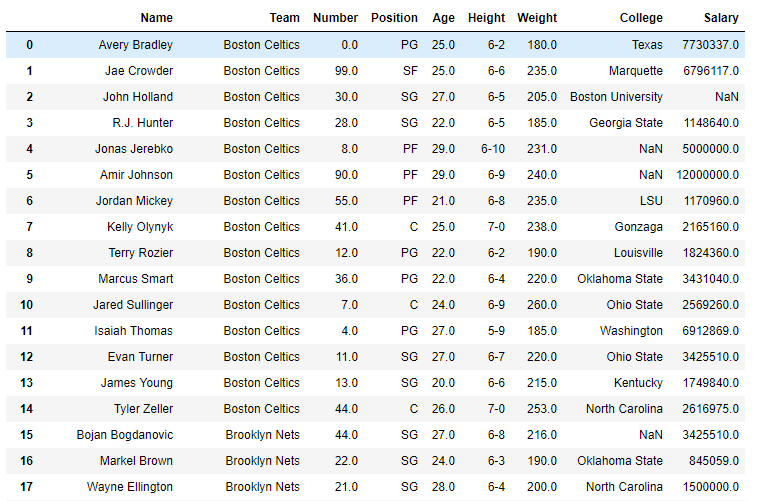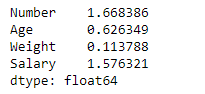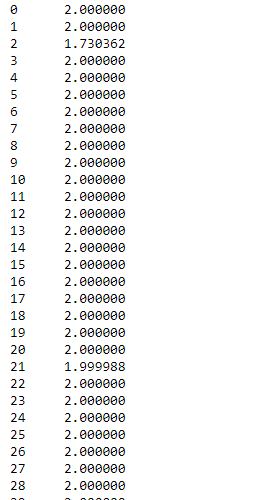Skip to content
Related Articles
Python | Pandas dataframe.skew()
• Last Updated : 19 Feb, 2021

Python is a great language for doing data analysis, primarily because of the fantastic ecosystem of data-centric python packages. Pandas is one of those packages and makes importing and analyzing data much easier.

Pandas` dataframe.skew()` function return unbiased skew over requested axis Normalized by N-1. Skewness is a measure of the asymmetry of the probability distribution of a real-valued random variable about its mean. For more information on skewness, refer this link.

Pandas: DataFrame.skew(axis=None, skipna=None, level=None, numeric_only=None, **kwargs)

Parameters :
axis : {index (0), columns (1)}
skipna : Exclude NA/null values when computing the result.
level : If the axis is a MultiIndex (hierarchical), count along a particular level, collapsing into a Series
numeric_only : Include only float, int, boolean columns. If None, will attempt to use everything, then use only numeric data. Not implemented for Series.

Return : skew : Series or DataFrame (if level specified)

For link to the CSV file used in the code, click here

Example #1: Use `skew()` function to find the skewness in data over the index axis.

 `# importing pandas as pd``import` `pandas as pd`` ` `# Creating the dataframe ``df ``=` `pd.read_csv(``"nba.csv"``)`` ` `# Print the dataframe``df`Let’s use the `dataframe.skew()` function to find skewness

 `# skewness along the index axis``df.skew(axis ``=` `0``, skipna ``=` `True``)`

Output :Example #2: Use `skew()` function to find the skewness of the data over the column axis.

 `# importing pandas as pd``import` `pandas as pd`` ` `# Creating the dataframe ``df ``=` `pd.read_csv(``"nba.csv"``)`` ` `# skip the na values``# find skewness in each row``df.skew(axis ``=` `1``, skipna ``=` `True``)`

Output :Attention geek! Strengthen your foundations with the Python Programming Foundation Course and learn the basics.

To begin with, your interview preparations Enhance your Data Structures concepts with the Python DS Course. And to begin with your Machine Learning Journey, join the Machine Learning – Basic Level Course

My Personal Notes arrow_drop_up